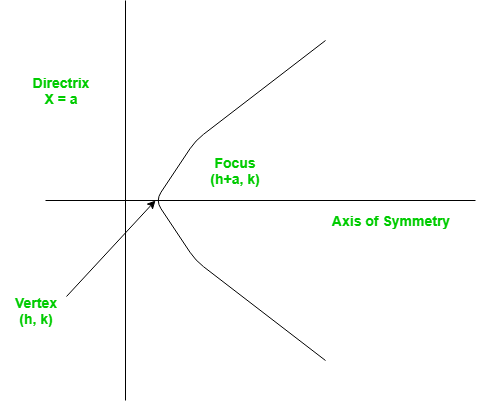C/C++ Program for Finding the vertex, focus and directrix of a parabola

A set of points on a plain surface that forms a curve such that any point on that curve is equidistant from the focus is a parabola.
Vertex of a parabola is the coordinate from which it takes the sharpest turn whereas a is the straight line used to generate the curve.The standard form of a parabola equation is. Given the values of a, b and c; our task is to find the coordinates of vertex, focus and the equation of the directrix.

Example –

Input : 5 3 2
Output : Vertex:(-0.3, 1.55)
Focus: (-0.3, 1.6)
Directrix: y=-198
Consult the formula below for explanation.

Recommended: Please try your approach on {IDE} first, before moving on to the solution.

 #include using namespace std;    // Function to calculate Vertex, Focus and Directrix void parabola(float a, float b, float c) {     cout << "Vertex: (" << (-b / (2 * a)) << ", "          << (((4 * a * c) - (b * b)) / (4 * a))          << ")" << endl;     cout << "Focus: (" << (-b / (2 * a)) << ", "          << (((4 * a * c) - (b * b) + 1) / (4 * a))          << ")" << endl;     cout << "Directrix: y="          << c - ((b * b) + 1) * 4 * a << endl; }    // Driver Function int main() {     float a = 5, b = 3, c = 2;     parabola(a, b, c);     return 0; }

Output:

Vertex: (-0.3, 1.55)
Focus: (-0.3, 1.6)
Directrix: y=-198

Please refer complete article on Finding the vertex, focus and directrix of a parabola for more details!

My Personal Notes arrow_drop_up

Article Tags :

Be the First to upvote.

Please write to us at contribute@geeksforgeeks.org to report any issue with the above content.